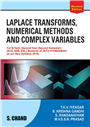Home > Higher Education > Mathematics > Differential Equations and Vector Calculus-(JNTU Anantapur)# Differential Equations and Vector Calculus-(JNTU Anantapur), 2/e

## Dr. T K V Iyengar, Dr. M.V.S.S.N. PRASAD, S. RANGANATHAM & DR. B. KRISHNA GANDHI

 ISBN : 9789352838264 Pages : 512 Binding : Paperback Language : English Imprint : S. Chand Publishing Trim size : 6.75" X 9.5" inches Weight : 0.4kg
 List Price :350.00
 Offer Price :280.00

20% OFF

# In Stock

Credit Card / Debit Card / Internet BankingThere exist many problems in engineering and science which are governed by linear or nonlinear ordinary differential equations. The two forms of linear differential equations with variable coefficients which can be reduced to linear differential equations with constant coefficients by using proper substitutions, has been discussed. The linear partial differential equations of second and higher order with constant coefficients. These can be divided into two groups, homogeneous and non-homogenous linear equations. In this book, how to solve such type equations has been elaborately described. In this book, vector differential calculus is considered, which extends the basic concepts of (ordinary) differential calculus, such as, continuity and differentiability to vector functions in a simple and natural way. This book comprises previous question papers problems at appropriate places and also previous GATE questions at the end of each chapter for the benefit of the students 1. Linear Differential Equations of Higher Order, 2. Equations Reducible to Linear Differential Equations, 3. Partial Differential Equations of First Order, 4. Partial Differential Equations of Higher Order, 5. Vector Differentiation and Vector Operators, 6. Vector Integration, 7. Vector Integral Theorems The two forms of linear differential equations with variable coefficients which can be reduced to linear differential equations with constant coefficients by using proper substitutions, has been discussed. The linear partial differential equations of second and higher order with constant coefficients. These can be divided into two groups, homogeneous and non-homogenous linear equations. In this book, how to solve such type equations has been elaborately described. In this book, vector differential calculus is considered, which extends the basic concepts of (ordinary) differential calculus, such as, continuity and differentiability to vector functions in a simple and natural way. Previous GATE questions at the end of each chapter for the benefit of the students. Questions with answers from latest question papers of JNTU A, have been inserted at proper places. The objective type questions have been given at the end of each unit### More Books By Author#### Laplace Transforms, Numerical ...

9789352838219450.00360.00

20% OFF#### Engineering Mathematics Vol. ...

9789352830305350.00280.00

20% OFF350.00280.00